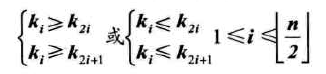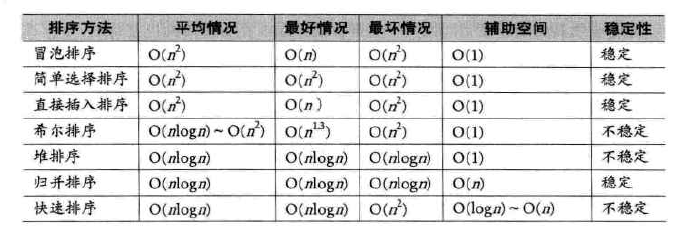1 篇文章 0 订阅

# 一、排序的基本概念与分类

### （3）内部排序与外部排序

• 时间性能：排序是数据处理中经常执行的一种操作，往往属于系统的核心部分，因此排序算法的时间开销是衡量其好坏的最重要的标志。在内部排序中，主要进行两种操作：比较和移动。比较是指关键字的比较，这是要做排序最起码的操作。移动指记录从一个位置移动到另外一个位置。高效率的内部排序算法应该是具有尽可能少的关键字比较次数和尽可能少的记录移动次数。

• 辅助空间：辅助存储空间是除了存放待排序所占用的存储空间之外，执行算法所需要的其他存储空间。

• 算法的复杂性：这里指的是算法本身的复杂度，而不是指算法的时间复杂度。显然算法过于复杂也会影响排序的性能。

### （4）排序中用到的函数

void swap(int *a, int i, int j)
{
int temp = a[i];
a[i] = a[j];
a[j] = temp;
} 

# 二、常用排序算法

### （1）冒泡排序

/*对数组a做交换排序（冒泡排序初级版）*/
void BubbleSort0(int *a, int n)
{
int i, j;
for(i = 1; i <= n; i++)
{
for(j = i+1; j <= n; j++)
{
if(a[i] > a[j])
swap(a, i, j);
}
}
} 

/*对数组a做交换排序（正宗版冒泡排序）*/
void BubbleSort(int *a, int n)
{
int i, j;
for(i = 1; i <= n; i++)
{
for(j = n-1; j >= i; j--)
{
if(a[j] > a[j+1])
swap(a, j, j+1);
}
}
} 

/*对数组a做交换排序（优化后冒泡排序）*/
void BubbleSort2(int *a, int n)
{
int i, j;
bool flag = true;
for(i = 1; i <= n && flag; i++)
{
flag = false;
for(j = n-1; j >= i; j--)
{
if(a[j] > a[j+1])
{
swap(a, j, j+1);
flag = true;
}
}
}
} 

### （2）简单选择排序

/*对数组a做简单选择排序*/
void SelectSort(int *a, int n)
{
int i, j, min;
for(i = 1; i < n; i++)
{
min = i;
for(j = i+1; j <= n; j++)
{
if(a[min] > a[j])
min = j;
}
if(i != min)
swap(a, i, min);
}
} 

### （3）直接插入排序

/*对顺序表a做直接插入排序*/
void InsertSort(int *a, int n)
{
int i, j;
int temp;
for(i = 2; i <= n; i++)
{
if(a[i] < a[i-1])
{
temp = a[i];
for(j = i-1; a[j] > a; j--)
{
a[j+1] = a[j];
}
a[j+1] = temp;
}
}
}

### （4）希尔排序

• ①如何让待排序的记录个数较少呢？很容易就能想到的就是将原本大量记录数的记录进行分组。分割成若干子序列，此时每个子序列待排序的记录个数就比较少了，然后在这些子序列内分别进行直接插入排序，当整个序列都基本有序时，注意只是基本有序时，再对全体记录进行一次直接插入排序。

• ②我们一直再提的“基本有序”到底是什么样子？所谓基本有序就是小的关键字基本在前面，大的基本在后面，不大不小的基本在中间，像{2,1,3,6,4,7,5,8,9}这样可以称为基本有序，但是{1,5,9,3,7,8,2,4,6}就不能称为基本有序，因为9在第三位，2在倒数第三位。

/*对数组a做希尔排序*/
void ShellSort(int *a, int n)
{
int i, j;
int increment = n;
int temp;
do
{
increment = increment/3+1;
for(i = increment+1; i < n; i++)
{
temp = a[i];
for(j = i-increment; j > 0 && temp > a[j]; j -= increment)
{
a[j+increment] = a[j];
}
a[j+increment] = temp;
}
}while(increment > 1)
}

### （5）堆排序①如何由一个无序序列构建成一个堆？
②如果在输出堆顶元素后，如何调整剩余元素成为一个新的堆？

/*对数组a做堆排序*/
void HeapSort(int *a, int n)
{
int i;
for(i = n/2; i > 0; i--)
{
}
for(i = n; i > 1; i--)
{
swap(a, 1, i);
}
}

/*本函数调整a[s]的关键字，使a[s...m]成为一个大顶堆*/
void HeapAdjust(int *a, int s, int m)
{
int temp, j;
temp = a[s];
for(j = 2*s; j <= m; j *= 2)
{
if(j < m && a[j] < a[j+1])
j++;
if(temp >= a[j])
break;
a[s] = a[j];
s = j;
}
a[s] = temp;
}

### （6）归并排序

/*将SR[s·····n]归并排序为TR1[s····t]*/
void MSort(int SR[], int TR1[], int s, int t)
{
int m;
int TR2[MAXSIZE+1];
if(s == t)
TR1[s] = SR[s];
else
{
m = (s+t) / 2;
MSort(SR, TR2, s, m);
MSort(SR, TR2, m+1, t);
Merge(TR2, TR1, s, m, t);
}
}

/*将有序的SR[i····m]和SR[m+1····n]归并为有序的TR[i···n]*/
void Merge(int SR[], int TR[], int i, int m, int n)
{
int j, k, l;
for(j = m+1, k = i; i <= m && j <= n; k++)
{
if(SR[i] < SR[j])
TR[k] = SR[i++];
else
TR[k] = SR[j++];
}
if(i <= m)
{
for(l = 0; i <= m-i; i++)
TR[k+i] = SR[i+1];
}
if(j <= n)
{
for(l = 0; l <= n-j; l++)
TR[k+l] = SR[j+l];
}
}

### （7）快速排序

/*对数组a的子序列a[low···high]做快速排序*/
void QSort(int * a, int low, int high)
{
int pivot;
if(low < high)
{
pivot = Partition(a, low, high);
QSort(a, low, pivot-1);
QSort(a, pivot+1, high);
}
}

/**/
int Partition(int *a, int low, int high)
{
int pivotkey;
pivotkey = a[low];
while(low < high)
{
while(low < high && a[high] >= pivotkey)
high--;
swap(a, low, high);
while(low < high && a[low] <= pivotkey)
low++;
swap(a, low, high);
}
return low;
}

# 三、总结• 简单算法：冒泡、简单选择、直接插入
• 改进算法：希尔、堆、归并、快速排序

10-163308-082万+
08-2448
03-299万+
05-22
03-13
07-271208
12-03141
07-191万+
11-06
07-15
04-053260
06-074万+点击重新获取扫码支付余额充值